Inconsistency in the Development of the Lorentz Factor in Section 3 of and#8216;On the Electrodynamics of Moving Bodiesand#8217; | OMICS International
Journal of Physical Mathematics
All submissions of the EM system will be redirected to Online Manuscript Submission System. Authors are requested to submit articles directly to Online Manuscript Submission System of respective journal.

# Inconsistency in the Development of the Lorentz Factor in Section 3 of ‘On the Electrodynamics of Moving Bodies’

Masahiko Makanae*

Representative Free Web College, Nishikasai, Edogawa-ku, Tokyo 134-0088, Japan

*Corresponding Author:
Makanae M
Independent Researcher
Representative Free Web College
Nishikasai, Edogawa-ku
Tokyo 134-0088, Japan
Tel:81-(0)3-3878-9542
E-mail: [email protected]

Received Date: March 15, 2017; Accepted Date: March 29, 2017; Published Date: March 31, 2017

Citation: Makanae M (2017) Inconsistency in the Development of the Lorentz Factor in Section 3 of ‘On the Electrodynamics of Moving Bodies’. J Phys Math 8:219. doi: 10.4172/2090-0902.1000219

Copyright: © 2017 Makanae M. This is an open-access article distributed under the terms of the Creative Commons Attribution License, which permits unrestricted use, distribution, and reproduction in any medium, provided the original author and source are credited.

Visit for more related articles at Journal of Physical Mathematics

#### Abstract

In 1905, Albert Einstein published his paper ‘On the Electrodynamics of Moving Bodies’, which is referred as the special theory of relativity. In Section 3 of this work, he developed a factor for describing a moving system in terms of a reference stationary system known as the Lorentz factor. In the climax of the development of Lorentz factor in this section, Einstein provided the series of equations that contains the mutual terms c, v, t, and x’. Clearly, ‘c’ corresponds to the velocity of light, ‘v’ is the velocity of the moving system, and ‘t’ is the time passage in the reference stationary system. However, regarding x’, the meaning is not clear as a mutual term. Besides, the values of x', which should have identical values, differ for the various equations. Thus, there is an inconsistency as regarding the usage of x' in the development of the Lorentz factor in Section 3 of ‘On the electrodynamics of moving bodies’. This issue should be discussed not only from the viewpoint of mathematics, but also from the viewpoint of formal logic, which is a practical tool for the study of any science.

#### Keywords

Galilean transportation; Lorentz factor; Contradiction; Einstein

#### Introduction

In 1905, Albert Einstein published his paper ‘On the Electrodynamics of Moving Bodies’  (the German original title is ‘Zur Elektrodynamik bewegter Körper’ ), which is referred to as the special theory of relativity (STR). In Section 1 of that paper, Einstein defines the concept of ‘time’, and then describes a method of confirming the synchronization of two clocks placed at two points. In Section 2 , based on ‘the principle of relativity’ and ‘the principle of the constancy of the velocity of light’, Einstein considers the relationships between a moving system and a reference stationary system with the concepts of ‘length’ and ‘time’, by using the universal equation ‘time=distance/ velocity’. Both systems have a relationship in the situation that an observer at rest in the reference stationary system observes an event in the moving system, which moves under parallel translation with uniform velocity with respect to the reference stationary system. Through the consideration in Section 2 , Einstein implies that an event in a moving system viewed within that moving system differs from the same event viewed from a reference stationary system. This perspective became the fundamental basis of the STR.

In Section 3 of the paper , Einstein develops the expression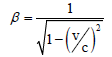which was later termed the ‘Lorentz factor’, as a core theory of the STR. This expression denotes the ratio of the values of ‘time’ or ‘length’ between the moving system and the reference stationary system when both systems are in the situation described above. The expression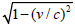in the Lorentz factor denotes the condition of length or time in the moving system viewed from the reference stationary system, when the reference value of length or time in the stationary system is defined to be unity. In this expression, c denotes the velocity of light, and v denotes the velocity of the moving system.

For this development, he assumed or implied the following conditions.

1. K is a reference stationary system.

2. k is another system in parallel with K.

3. k moves at a uniform velocity along the x-axis of K in the positive direction; this velocity is denoted as v.

4. t is time passage in K.

5. τ is time passage in k.

6. c is the velocity of light. (Note: In the German original text , the velocity of light is denoted as ‘V’.)

7. A light source and a clock are placed at the origin of k.

8. A mirror and another clock are placed at a specific point of the x-axis of k.

9. A light source emits a ray of light in the positive direction of the x-axis of k and K, when t=0.

10. ‘For brevity, at the origin of k, τ=0, when t=0’.

11. Confirming the synchronization of the two clocks, by using a round trip of the ray between the two clocks. This method was previously established and examined in Sections 1 and 2 .

12. The ray of light obeys ‘the principle of the constancy of the velocity of light’, which defined as ‘Any ray of light moves in the “stationary” system of co-ordinates with the determined velocity c, whether the ray be emitted by a stationary or by a moving body.’ in Section 2 .

13. ‘To any system of values x, y, z, t, which completely defines the place and time of an event in the stationary system, there belongs a system of values ξ, η, ζ, τ, determining that event relatively to the system k’.

14. In the process of the development of Lorentz factor, Einstein provides the following equation as an initial equation in the description: ‘If we place x'=x−vt, it is clear that a point at rest in the system k must have a system of values x', y, and z, independent of time.’

x'=x–vt. (1)

He then provides many equations, before providing the following equation in the part before the first appearance of Lorentz factor in Section 3 .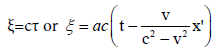(2)

The term ‘ξ’ of the equation ‘ξ=cτ’ corresponds to ‘distance’ of the universal equation: ‘distance=velocity × time’. The term ‘a’ in equation (2) was described as ‘function φ (v) at present unknown’; ‘function φ (v)’ is revealed φ (v)=1 before the second and also the last appearance of the Lorentz factor in Section 3 . In other words, a=1.

Then, Einstein provides the following equation in the description, which we will term Sentence 1: ‘But the ray moves relatively to the initial point of k, when measured in the stationary system, with the velocity c−v, so that: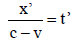(3)

Then he describes ‘If we insert this value of t in the equation for ξ, we obtain: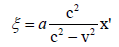(4)

The fractional expression, i.e., the denominator and numerator in the right-hand side of equation (4) has the same structure as the lefthand side of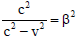which was provided in Section 4 of the paper ‘Electromagnetic phenomena in a system moving with any velocity smaller than that of light’  by Lorentz himself published in 1904., can be factorized to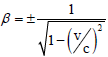.

After equation (4), in total five equations and two sets of equations were provided, then the form, appears for the first time . As described in the above, the term x' is contained in the initial equation (1), and also in the series of equations (2), (3) and (4) that configure the final stage of the development of Einstein’s Lorentz factor. Explanations or definitions are not provided as a sentence for each x', while the values of x' of equations (1) or (3) are clearly provided or implied as a formula. Thus, it is natural to interpret that the meaning of x' of equations (1), (2), (3), and (4) are the same. However, if examining each equation by using numerical values of practical example, we find that the meanings or tangible values of x' of equations (1), (2), (3), and (4) are different. Thus, we can say that there is an inconsistency as regarding the usage of x' in the development of Lorentz factor in Section 3 . In this paper, we explain the details of this issue, and then suggest the solution to avoid any doubt that the grounds of Lorentz factor are unclear.

Establishment of the conditions

We assume mutual conditions for examining x' of equations (1), (2), (3), and (4); but, before assuming the conditions, we should define the concept of ‘point’ and ‘position’, because the key term x' or x may correspond to ‘point’ or ‘position’ in , whereas Einstein did not provide a definition of ‘point’ or ‘position’ as an independent description focus in each term. Probably, he thought that both terms are popular; thus, even a non-scientist can understand each meaning without definition. However, ‘point’ and ‘position’ are fundamental concepts that must be established in order to consider the relationship between K and k, thus we provide the following definitions for proceeding with our study, except for the case of using the term ‘point’ in the phrase ‘time point’ or ‘point in time’.

• ‘point’ is: A stationary location on the x-axis of k.

• ‘position’ is: A location of a certain point viewed from K. It may move with k viewed from K.

Further, we assume that the value of ‘point’ can be obtained when measuring it by using a ruler fixed on the x-axis of k (ruler of k) and the value of ‘position’ can be obtained when measuring it by using a ruler fixed on the x-axis of K (ruler of K).

Now, we assume numerical values of a practical example as the mutual conditions based on the conditions 1 to 13 described in the previous section.

• v is the half of the velocity of light, i.e., 0.5 c.

• The distance between the light source and the mirror is 1l viewed within k.

• Light moves 1l in 1 s.

Examination of x' of equation (1)

The structure of equation (1) is identical to Galilean transportation, namely x'=x–vt, whereas Einstein does not state so in Section 3 . This equation denotes that a point, which moves with v viewed from K, is at rest always when viewed within k. In other words, the value of x' of equation (1) is constant as it is the value of the distance between the origin of k and point x' viewed within k.

Now, let us examine x' of equation (1). For this purpose, we denominate the point where the mirror is placed, as x'; and we denominate the variable position moving along the x-axis of K with the location of x' viewed from K, as x. By using the numerical values of practical example, we can assume the following tangible situation.

1. The distance between the origin of k and x' is always 1l measured by the ruler of k.

2. The value of the distance from x' at 0 s to x after the light source had emitted the ray increases; it can be calculated by vt.

3. When x'+vt=ct, i.e., when 1l+vt=ct, the mirror reflects the front of the ray (i.e., the tip of the ray). 1l+vt=ct can be transposed to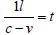.

4. By inserting the numerical value to, we obtain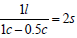.

From the above, we confirm

x'=1l. (5)

As shown above, v or t does not affect the value of x'; in other words, the conditions 2, 3, and 4 of the above situation do not affect the value of x'. This is natural because the value of x' of equation (1) is constant in the first place. Therefore, if changing the value of v to more or less than 0.5 c, or changing the value of t to more or less than 2 s, the value of x' is still the same.

Examination of x' of equation (2)

In Section 3 , after providing the set of equations ξ=cτ or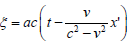and the equation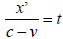, Einstein describes ‘If we insert this value of t in the equation for ξ, we obtain’ and then provides equation (4). The phrase ‘this value of t’ corresponds to the formula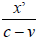. The phrase ‘the equation for ξ’ actually corresponds to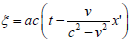, not ξ=cτ. Therefore, hereafter, we employ only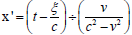as equation (2) for convenience. Under the premise that a=1 as Einstein implied, equation (2) can be transposed(6)

If we assume that equation (1) affects the whole process of the development of the Lorentz factor, x' of (2) must obey the initial equation (1), thus the value of x' of (6) must be 1l. In other words,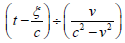must be 1l.

However, this formula, which can be described as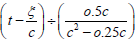by inserting the numerical value of v, cannot provide 1l. Besides, equation (6) corresponds to a structure of ‘distance=time ÷ velocity ÷ velocity’ or ‘distance=time ÷ a ratio of velocities’ (because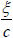provides ‘time’), thus it does not match with the universal equation time=distance/velocity, which is used as the fundamental theory in the discussion of Sections 1 and 2 of , and thus it does not provide any value of ‘distance’ or ‘time’ or ‘velocity’.

Examination of x' of equation (3)

Equation (3), namely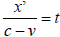, can be transposed to x'=ct–vt; thus, the method of calculating the value of x' is very clear. However, the meaning of x' of equation (3) is not clear, because there is ambiguity in the meaning of ‘the initial point of k’ in terms of whether it is variable or a constant. This is because:

The term ‘the initial point of k’ is provided as the one of premises ofin Sentence 1, i.e., ‘But the ray moves relatively to the initial point of k, when measured in the stationary system, with the velocity c–v, so that.’

The value ‘ct’ in ‘x'=ct–vt’, which can be extracted from.

The value ‘ct’ implies that the value of the distance between the position of the origin of k at a point in time at which the light source emits the ray and the front of the ray at a point in time at which the observer at rest in the reference stationary system measures the position of the front of the ray.

• The term ‘the initial point of k’ is provided as the one of premises of in Sentence 1, i.e. ‘But the ray moves relatively to the initial point of k, when measured in the stationary system, with the velocity c–v, so that.’

• The value ‘ct’ in ‘x'=ct–vt’, which can be extracted from.

The value ‘ct’ implies that the value of the distance between the position of the origin of k at a point in time at which the light source emits the ray and the front of the ray at a point in time at which the observer at rest in the reference stationary system measures the position of the front of the ray.

• The value ‘vt’ of ‘x'=ct–vt’ implies the value of the distance between the position of the origin of k at a point in time at which the light source emits the ray and the position of the origin of k at a point in time at which the observer at rest in the reference stationary system measures this position.

• The formula ‘ct–vt’ can be factored as ‘(c–v)t’; thus, we can say that ‘c–v’ indicates the relative velocity between the front of the ray and the position of the origin of k.

• Based on the above assumptions, we can interpret the sentence ‘the ray moves relatively to the initial point of k’ to mean that the front of the ray moves relative to the position of the origin of k.

• From the above, we can say that ‘the initial point of k’ corresponds to ‘the position of the origin of k’, which varies with vt viewed from the reference stationary system.

• However, the term ‘initial’ give us the impression that it has a constant value from the time it had occurred.

Thus, we should clearly define ‘the initial point of k’, before examining x' of equation (3).

The English term ‘initial point of k’ is extracted by referring to the English-translated version . Indeed, in Section 3 , similar terms ‘the origin of k’ and ‘the origin of system k’ are provided together with ‘the initial point of k’. Further, ‘the origin of one of the two systems (k)’, ‘the initial position of the moving system’, and ‘the origin of co-ordinates’ are described without thorough explanations of the differences between them. Thus, let us confirm the original German descriptions. Below, the former (left side) is the original German text described , and the latter (right side) is the English-translated text of the paper .

1. ‘Anfangspunkte von k’ to ‘the origin of k’

2. ‘Anfangspunkt von k’ to ‘the initial point of k’

3. ‘Anfangspunkt des Systems k’ to ‘the origin of system k’

4. ‘dem Anfangspunkte des einen der beiden Systems (k)’ to ‘the origin of one of the two systems (k)’

5. ‘Anfangslage des bewegten Systems’ to ‘the initial position of the moving system’

6. ‘dem Koordinatenursprunges’ to ‘the origin of co-ordinates’

In the literal translation; the German term ‘Anfang’ means ‘beginning’, ‘punkt’ or ‘punkte’ means ‘point’, and ‘lage’ means ‘situation’, but it can be reasonably translated as ‘position’. The German term ‘s’ in ‘Anfangspunkt’ or ‘Anfangslage’ has the role of connecting the former and the latter terms. Therefore, we can say that in the literal translation ‘Anfangspunkt’ (or ‘Anfangspunkte’) is the ‘beginning point’. Likewise, ‘Anfangslage’ is the ‘beginning position’. However, the German term ‘Anfang’ had been translated as ‘initial’ or ‘origin’ as shown in translations 1, 2, 3, 4, and 5 above. On the other hand, in translation 6, the literal translation ‘the origin of co-ordinate’ had been employed for ‘dem Koordinatenursprunges’ (‘Koordinaten’ corresponds to ‘co-ordinates’ and ‘Ursprung’ corresponds to ‘origin’).

It seems to be slightly complicated to be comparing the English terms with the original German terms and the literally translated terms; especially for the reason that ‘Anfang’ (i.e., ‘beginning’ as the literal translation) is translated in two ways as ‘initial’ and ‘origin’ . However, from the view point of a time sequence, we can arrange the above phrases as below.

First, we treat all references such as ‘the origin of one of the two systems (k)’ and ‘the origin of system k’ as references to ‘the origin of k’ and assume that ‘the origin of k’ has the absolute value as the zero point of x, y, z-axes of k. However, k is in motion as viewed from K; thus, the position of ‘the origin of k’ is variable, as viewed from K. Regarding the phrase: ‘the initial position of the moving system’, we assume that this corresponds to the position of the origin of k at the point in time at which the light source emits the ray as viewed from K. It is natural that this value is not variable; this is because the light source emits the ray at a definite time 0 s.

On the basis of the above arrangement, we define ‘the initial point of k’ as the position of the origin of k at a point of time where the observer at rest in the stationary system measures the distance between the origin of K and this position by using the ruler of K, whereas the English term ‘initial’ still give us the impression that it has a constant value. Thus, we state that x' of x'=ct–vt denotes the value of the distance between the front of the ray and ‘the initial point of k’ measured by the ruler of K, and ‘the velocity c–v’ is the relative velocity between the front of the ray and ‘the initial point of k’. If it is difficult to use the term ‘initial’ despite the above being an accepted definition, we suggest employing the literally translated term ‘beginning point’ instead of ‘initial point’, otherwise unifying the translations of ‘Anfangspunkte von k’ (described as ‘the origin of k’ ) and ‘Anfangspunkt von k’ (described as ‘the initial point of k’ ) as ‘the origin of k’.

With the above, we conclude the confirmation of the terms, and we now return to the main subject. If equation (1) is provided as the initial equation that affect the whole process of development of Lorentz factor, then the meaning and the value of x' of (1) and (3) must be the same. If the meaning and the value of x' of (1) and (3) are the same, we can substitute the value of x' of (1) to x' of (3), then (3) becomes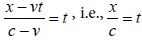. The form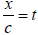corresponds to the universal equation distance/velocity=time. This means that the relative velocity between the front of the ray and ‘the initial point of k’, namely ‘ c-v’, is cancelled. At the same time, the term ‘v’, which is required as an element of Lorentz factor, is cancelled. Therefore, it is not appropriate to treat x' of equation (1) and x' of equation (3) together in the same context in the development of the Lorentz factor.

Next, we calculate the value of x' of equation (3) by using the numerical values of the practical example. Under the premise that x' of equation (3) corresponds to the distance between the front of the ray and ‘the initial point of k’ viewed from K, we can assume the following situation.

1. The value of the distance between the front of the ray and ‘the initial point of k’ increases with ct–vt measured by the ruler of K.

2. At the time point when the light source emits the ray (i.e., when t=0), the value of the distance from ‘the initial point of k’ to the mirror is 1l measured by the ruler of K. This value is the same as the value of the distance between the light source and the mirror viewed within k.

3. The time interval from the time point when the light source emits the ray to the time point when the mirror reflects the front of the ray in K; it can be calculated by using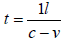. Because it was previously confirmed that the mirror reflects the front of the ray when 1l+vt=ct as viewed from K, 1l+vt=ct can be transposed to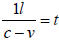. Inserting the numerical value of v to, we obtain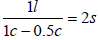. Thus, this time interval is 2 s.

From the above, we can say that the value of x' of equation (3) at the time point when the mirror reflects the front of the ray viewed from K is 1l, i.e.,

x'=1l. (7)

Here, we find that the two different x', namely x' of equation (1) and x' of equation (3), have the same value as shown as equations (5) and (7) are exactly the same in the tangible situation assumed in our study. At a glance, it seems that even the meaning of x' of equations (1) and (3) are different, but both values are always the same. However, if assuming the other situation that putting a completely transparent glass plate instead of the mirror and more than 2 s (for example 20 s) passes after the light source emitted the ray, we obtain x'=10l for (3). In this situation, the two different x' have the two different values 1l in equation (1) vs. 10l in equation (3). It is natural that we obtain this difference, because the value of x' of equation (1) is constant as 1l, even if the time passes 20 s. However, the time passage is not the original cause of this difference. The original cause is the act of using the transparent glass instead of the mirror. Under this condition the ray goes through the glass plate then advances only in the positive direction of the x-axis, thus the value of ct–vt (i.e., the value of x') increases with no limit. If precluding the glass plate then putting the mirror back, the mirror reflects the front of the ray at 2 s; thus the value of ct–vt (i.e., the value of x') cannot increase after 2 s. In other words, the value of the distance of the trip of the ray in the positive direction of x-axis is 1l viewed within k or 2l viewed from K, even if the time passes more than 2 s.

From this fact, we can say that Einstein's Sentence 1 does not match with his STR that requires confirming the synchronization of the two clocks by using the round trip of the ray. Because, Sentence 1 does not contain a condition such as ‘until the mirror reflects the ray’, which is necessary in order to perform the round trip of the ray. In other words, the one of the essential conditions of STR is lacking in Sentence 1.

Paper  is referred to as Einstein’s special theory of relativity, and many people interpret that ‘special’ of ‘the special theory of relativity’ implies the special condition regarding the relationship between the moving system and the reference stationary system that the former moves with uniform velocity along the x-axis of the latter in parallel translation. This interpretation itself is correct; however, the light source, the mirror (in order to reflect the ray), and the round trip between two clocks for confirming the synchronization of the two clocks, are also the special conditions that are required in order to establish Einstein's STR. In other words, if one of these special conditions is lacking, his STR cannot hold.

Examination of x' of equation (4)

Equation (4), namely,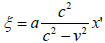, is obtained by substituting the value of t of (3) to t of (2). We previously confirmed that a=‘function φ (v)=1’. Therefore, we can reduce (4) to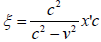. We can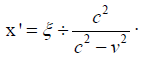If we assume that equation (1) affects the whole process of the development of the Lorentz factor, x' of equation (8) must obey the initial equation (1), thus the value of x' of (8) must be 1l. In other words,must be 1l. However, this formula, which can be described as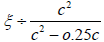by inserting the numerical value of v, cannot provide 1l. Besides, equation (8) corresponds to a structure of distance=distance ÷ velocity ÷ velocity or ‘distance=distance ÷ a ratio of velocities’, thus it does not match with the universal equation time=distance/velocity, which is used as the fundamental theory in the discussion of Sections (1) and (2) , and thus it does not provide any value of ‘distance’ or ‘time’ or ‘velocity’.

#### Overview

The velocity of light is described as V in the German original article ; on the other hand, it described as c in the Lorentz's article . However, for us who have read [1,3] in English, the Lorentz factor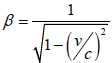is substantially implied at the stage where equation (4), which contains the left-hand side of Lorentz's equation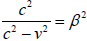in Section 4 , is provided. In other words, the series of equations (2), (3), and (4) configure the climax of the development of Lorentz factor .

The following is the expansion process that from (2), namely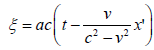, to (4), namely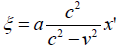by using the value of t of (3), namely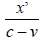.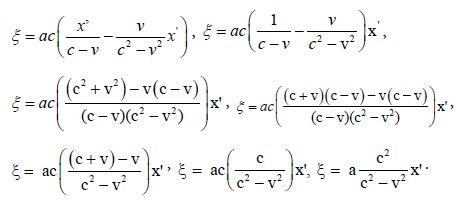As shown in the process from the first equation to the second equation in the above, the term x' has a role that simplifies the inside of the parenthesis by extracting x' of the left formula and x' of the right formula to the outside of the parenthesis. In the parenthesis of the first equation, the left formula is provided from equation (3), and the right formula is provided from equation (2). This means that the meaning and the value of x' of equations (2), (3), and (4) must be the same. Likewise, under the assumption that x' of equation (1) affects the whole process of the development of Lorentz factor, x' of (1) must be the same as x' of equations (2), (3), and (4). This perspective is correct not only from the viewpoint of mathematics but also from the viewpoint of the principle of identity provided by formal logic, which is a practical tool for the study of any science, including theoretical physics.

However,

• x' of (1) is constant, x' of equation (3) is variable.

• The meanings of x' of equations (2) and (4) are not clear.

• The value of x' of equations (2) or (4) differs from the value of x' of equations (1) or (3).

#### Conclusion

From the above, we can conclude that the equations (1), (2), (3), and (4) or some of these are not appropriate for the establishment of Lorentz factor; in other words, the development process of the Lorentz factor provided in Section 3  is not correct.

In contrast, including the indirect evidence provided through discoveries of our universe by using the general theory of relativity (GTR), which was developed in 1915, much evidence that proves the correctness of the Lorentz factor exists. If we ignore this contradiction and continue to use the Lorentz factor in order to study relativity, then modern science, which espouses Einstein’s STR, may at some point suffer from a lack of trust; therefore, this contradiction should be solved.

One of the ways to resolve the contradiction is to disprove the evidence; however, it may be difficult to proceed in this manner, because the evidence has been reported by highly trusted institutions and scientists. The other way is to state that the Lorentz factor was already established in ref.  before refs. [1,2] as a universal theory, whether Lorentz himself had recognized that his theory can be used at both the microscopic and the astronomical scale. However, this may also be unacceptable, because Einstein’s Lorentz factor was not established on the Lorentz’s article . In fact, Einstein did not discuss or introduce  in the development process of his Lorentz factor [1,2]. We know that Einstein provided a different method to derive the Lorentz factor in his book entitled ‘The Special and General Theory of Relativity’  published in 1916; but, if employing this method, we have to state that Einstein developed his Lorentz factor, which is a core theory of the STR, after releasing the GTR, which was developed based on STR.

It seems that there is no way to solve the contradiction. However, if we assume that the Lorentz factor is established as a categorical judgement, then the contradiction is simply removed. Because, if it is so, the Lorentz factor can be used as an absolute and universal theory that is never affected by any condition such as ‘until the mirror reflects the ray’, which is lacking in Sentence 1. Likewise, any development process is not necessary. Metaphorically speaking, it is appropriate to treat the Lorentz factor as the same as the equation 1+1=2 in the decimal system of mathematics, which holds without any explanation. Once this methodology has been employed, the above contradiction is removed, and we can avoid any doubt that the grounds of the Lorentz factor are unclear. In addition, under the assumption that the Lorentz factor is determined by categorical judgement, expressions describing the Lorentz factor as ‘a result of STR’ should not be used because such expressions imply that the concept of the Lorentz factor holds in certain premises, i.e., the Lorentz factor is not determined by categorical judgement. Therefore, we have used the phrase, ‘a core theory of STR’, on multiple occasions in our study.

In any case, the inconsistency in Section 3 [1,2] and the above methodology should be widely discussed, if we continue to use the Lorentz factor in the study of relativity.

#### Acknowledgments

I would like to thank editor for English language editing, Ms. Maxie Pickert for confirming the meanings of the German original text , Mr. Makoto Kawahara for confirming the basic theory of mathematics, and Ms. Noriko Teramoto for help with the English grammar.

#### References

Select your language of interest to view the total content in your interested language

### Article Usage

• Total views: 856
• [From(publication date):
March-2017 - Nov 18, 2019]
• Breakdown by view type
• HTML page views : 782Can't read the image? click here to refresh